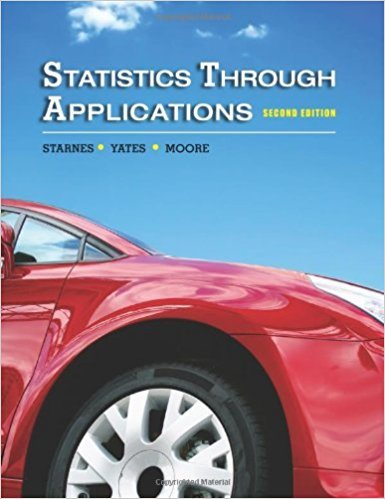×
×

# Solutions for Chapter 7: Probability: What Are the Chances?## Full solutions for Statistics Through Applications | 2nd Edition

ISBN: 9781429219747Solutions for Chapter 7: Probability: What Are the Chances?

Solutions for Chapter 7
4 5 0 343 Reviews
19
3
##### ISBN: 9781429219747

Chapter 7: Probability: What Are the Chances? includes 88 full step-by-step solutions. Since 88 problems in chapter 7: Probability: What Are the Chances? have been answered, more than 14450 students have viewed full step-by-step solutions from this chapter. This expansive textbook survival guide covers the following chapters and their solutions. This textbook survival guide was created for the textbook: Statistics Through Applications, edition: 2. Statistics Through Applications was written by and is associated to the ISBN: 9781429219747.

Key Statistics Terms and definitions covered in this textbook
• Attribute

A qualitative characteristic of an item or unit, usually arising in quality control. For example, classifying production units as defective or nondefective results in attributes data.

• Bimodal distribution.

A distribution with two modes

• Bivariate distribution

The joint probability distribution of two random variables.

• Block

In experimental design, a group of experimental units or material that is relatively homogeneous. The purpose of dividing experimental units into blocks is to produce an experimental design wherein variability within blocks is smaller than variability between blocks. This allows the factors of interest to be compared in an environment that has less variability than in an unblocked experiment.

• Central composite design (CCD)

A second-order response surface design in k variables consisting of a two-level factorial, 2k axial runs, and one or more center points. The two-level factorial portion of a CCD can be a fractional factorial design when k is large. The CCD is the most widely used design for itting a second-order model.

• Comparative experiment

An experiment in which the treatments (experimental conditions) that are to be studied are included in the experiment. The data from the experiment are used to evaluate the treatments.

• Confounding

When a factorial experiment is run in blocks and the blocks are too small to contain a complete replicate of the experiment, one can run a fraction of the replicate in each block, but this results in losing information on some effects. These effects are linked with or confounded with the blocks. In general, when two factors are varied such that their individual effects cannot be determined separately, their effects are said to be confounded.

• Conidence coeficient

The probability 1?a associated with a conidence interval expressing the probability that the stated interval will contain the true parameter value.

• Contingency table.

A tabular arrangement expressing the assignment of members of a data set according to two or more categories or classiication criteria

• Control limits

See Control chart.

• Cumulative sum control chart (CUSUM)

A control chart in which the point plotted at time t is the sum of the measured deviations from target for all statistics up to time t

• Defect concentration diagram

A quality tool that graphically shows the location of defects on a part or in a process.

• Discrete uniform random variable

A discrete random variable with a inite range and constant probability mass function.

• Empirical model

A model to relate a response to one or more regressors or factors that is developed from data obtained from the system.

• Enumerative study

A study in which a sample from a population is used to make inference to the population. See Analytic study

• Exhaustive

A property of a collection of events that indicates that their union equals the sample space.

• Fixed factor (or fixed effect).

In analysis of variance, a factor or effect is considered ixed if all the levels of interest for that factor are included in the experiment. Conclusions are then valid about this set of levels only, although when the factor is quantitative, it is customary to it a model to the data for interpolating between these levels.

• Forward selection

A method of variable selection in regression, where variables are inserted one at a time into the model until no other variables that contribute signiicantly to the model can be found.

• Gamma random variable

A random variable that generalizes an Erlang random variable to noninteger values of the parameter r

• Goodness of fit

In general, the agreement of a set of observed values and a set of theoretical values that depend on some hypothesis. The term is often used in itting a theoretical distribution to a set of observations.

×